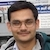# NAEST 2017 Screening Test: Water Rotating in a BucketBy

A bucket is filled with water upto a certain height. The water is set into rotation about the vertical axis. The surface of rotating water takes parabolic shape. Let us explain this with the help of Newton's laws of motion.

Question 1: When water is stationary, the force on the leaf due to water is

1. vertically upward
2. vertically downward
3. towards centre of the tub

Question 2: When water is rotating, the force on the leaf due to water is

1. vertically upward
2. vertically downward
3. towards centre of the tub (axis of rotation)
4. normal to the water surface (in contact with the leaf)

Answer: The acceleration of the leaf is zero when the water is stationary. Thus, by Newton's second law, the net force on the leaf is zero. The force on the leaf due to the water is vertically upward and it balances the weight.The leaf is rotating with an angular speed $\omega$ in a horizontal circle of radius $x$. The acceleration (centripetal) of the leaf is given by \begin{align} a=\omega^2 x. \end{align} The acceleration is in the horizontal plane and it always points towards the centre C. Thus, the direction of the net force on the leaf is towards the centre of the circle. The magnitude of the net force is $m\omega^2 x$.

Let the origin O of the coordinate system be at the intersection of the water surface and the axis of rotation. Let the leaf be at a point P($x,y$). The forces acting on the leaf are

1. its weight $mg$ in the downward direction.
2. force $F$ from the water in a direction normal to the water surface.
We assume tangential viscous force on the leaf to be negligibly small.Let the normal to the water surface at the point P($x,y$) make an angle $\theta$ with the vertical. It is easy to see that the tangent to the surface at P($x,y$) make the same angle $\theta$ with the horizontal i.e., \begin{align} \tan\theta=\frac{\mathrm{d}y}{\mathrm{d}x} \end{align}

Resolve force $F$ in the horizontal and the vertical directions and apply Newton's second law to get \begin{align} F\cos\theta&=mg \\ F\sin\theta&=m\omega^2 x. \end{align} Solve the above equations to get \begin{align} \mathrm{d}y=\frac{\omega^2}{g} x\mathrm{d}x \end{align} Integrate to get \begin{align} y=\frac{\omega^2}{2g} x^2. \end{align} This is an equation of a parabola. I encourage you to see the effect of $\omega$ on the shape of the parabola.